Function Repository Resource:

# NegativeCoordinateMarginalDistribution

Represent a MarginalDistribution permitting negative numbers as indices

Contributed by: Seth J. Chandler
 ResourceFunction["NegativeCoordinateMarginalDistribution"][dist,k] represents a univariate marginal distribution of the kth coordinate from the multivariate distribution dist, where k is allowed to be a negative index. ResourceFunction["NegativeCoordinateMarginalDistribution"][dist,{k1,k2,… }] represents a multivariate marginal distribution of the {k1,k2,…} coordinates, but extending the coordinate values to permit negative indices. ResourceFunction["NegativeCoordinateMarginalDistribution"][dist] represents the last marginal distribution of the distribution dist.

## Details

ResourceFunction["NegativeCoordinateMarginalDistribution"] determines the number of coordinates by considering the length of DistributionDomain applied to the distribution.
ResourceFunction["NegativeCoordinateMarginalDistribution"] can compute anything that MarginalDistribution can compute.

## Examples

### Basic Examples (2)

Get the marginal distribution of a CategoricalDistribution for its last coordinate:

 In:=Out=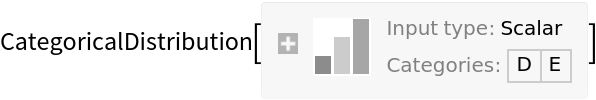Get the marginal distribution of a symbolic multivariate normal distribution for its penultimate coordinate:

 In:=In:=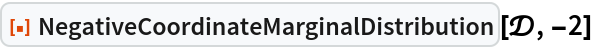Out=### Scope (2)

Positive and negative indices can be used together:

 In:=Out=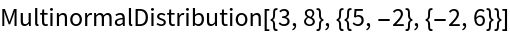If no index is specified, the last index is used:

 In:=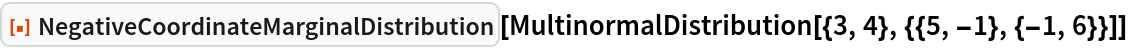Out=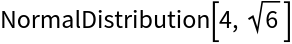### Applications (7)

A causal graph between anxiety, peer pressure and smoking:

 In:=Out=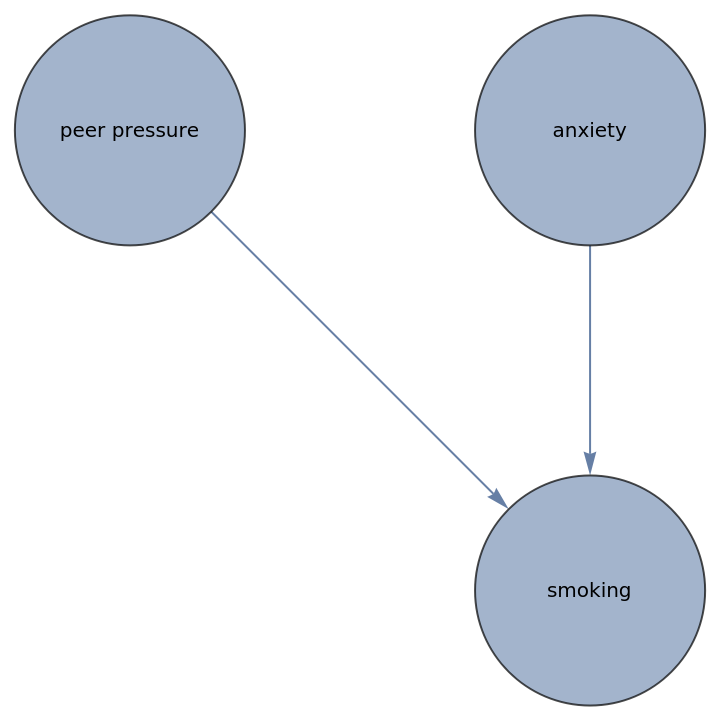Determine the effect of changing the probability of peer pressure on the probability of smoking as follows. First, generate categorical distributions for peer pressure and anxiety:

 In:=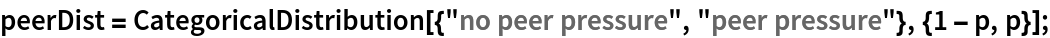In:=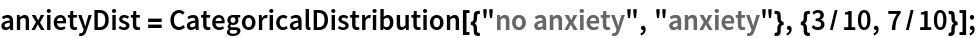Assume the two are independent and so one can find their joint distribution using the resource function MixtureCategoricalDistribution as follows:

 In:=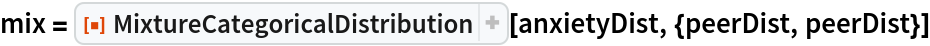Out=To use a CategoricalDistribution as input to another MixtureCategoricalDistribution, it must be univariate:

 In:=Out=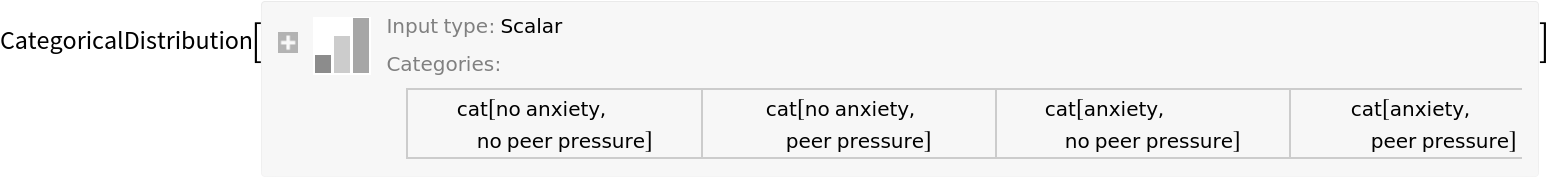Now form the distribution of smoking as a function of whether the person is anxious and subject to peer pressure:

 In:=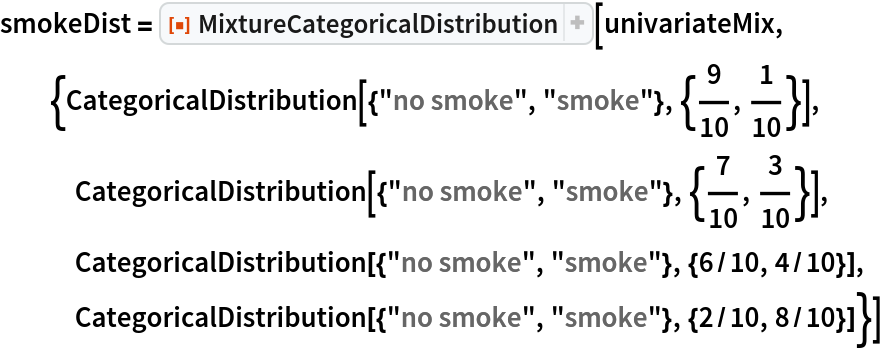Out=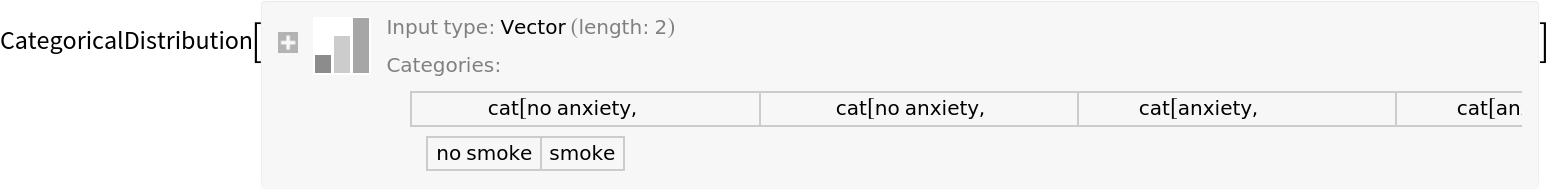Use NegativeCoordinateMarginalDistribution to get the probability of smoking as a function of the prevalence of peer pressure:

 In:=Out=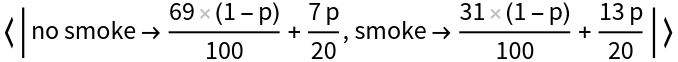Plot the relationship between the prevalence of peer pressure and the probability of smoking:

 In:=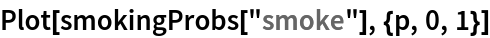Out=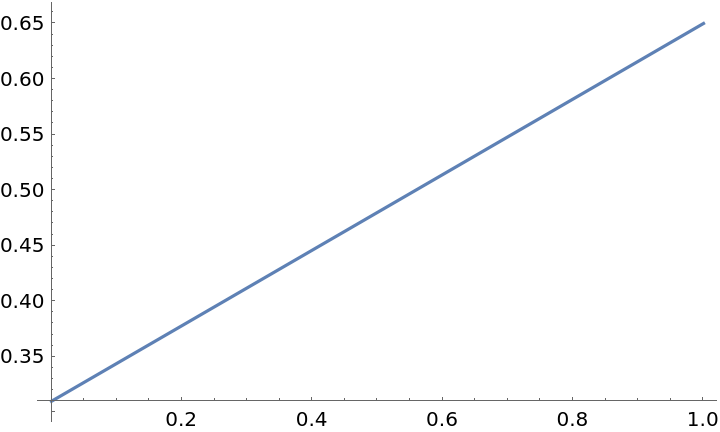### Properties and Relations (2)

NegativeCoordinateMarginalDistribution works the same as MarginalDistribution if a positive number is used for the index:

 In:=Out=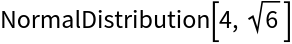In:=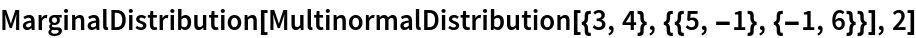Out=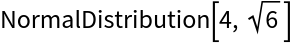NegativeCoordinateMarginalDistribution[dist] is equivalent to NegativeCoordinateMarginalDistribution[dist,-1]:

 In:=Out=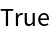Seth J. Chandler

## Version History

• 1.0.0 – 27 July 2021